• 这是一款以生产质量为核心的FIR滤波器设计，安全生产、质量生产成为了FIR滤波器设计主要内容，欢迎大家下...该文档为FIR滤波器设计，是一份很不错的参考资料，具有较高参考价值，感兴趣的可以下载看看
• 基于verilog的fir滤波器设计。在通信，数字信号领域使用非常广泛。
• [Matlab]FIR滤波器设计：(基本窗函数FIR滤波器设计) ​ IIR滤波器主要设计方法先设计一个模拟低通滤波器，然后把它转化为形式上的数字滤波器。但对于FIR滤波器来说，设计方法的关键要求之一就是保证线性相位条件。而...
[Matlab]FIR滤波器设计：(基本窗函数FIR滤波器设计)
​ IIR滤波器主要设计方法先设计一个模拟低通滤波器，然后把它转化为形式上的数字滤波器。但对于FIR滤波器来说，设计方法的关键要求之一就是保证线性相位条件。而IIR滤波器的设计方法中只对幅值特性进行设计，因此无法保证相位。所以FIR滤波器的设计需要采用完全不同的方法。FIR滤波器的设计方法主要有窗函数法、频率采样法、切比雪夫逼近法等。
​ 由于理想滤波器在边界频率处不连续，故时域信号

h

d

(

n

)

h_d(n)

一定是无限时宽的，也是非因果的。所以理想低通滤波器是无法实现的。如果实现一个具有理想线性相位特性的滤波器，其幅值特性只能采用逼近理想幅频特性的方法实现。如果对时域信号

h

d

(

n

)

h_d(n)

进行截取，并保证截取过程中序列保持对称，而且截取长度为N,则对称点为

α

=

1

2

∗

(

N

−

1

)

α=\frac{1}{2}*(N-1)

。截取后序列为

h

(

n

)

h(n)

，侧

h

(

n

)

h(n)

可用下式子表示：

h

(

n

)

=

h

d

(

n

)

∗

w

(

n

)

h(n) = h_d(n)*w(n)

式中，

w

(

n

)

w(n)

为截取函数，又称为穿函数。如果窗函数为矩形序列，则称之为矩形窗。窗函数有多种形式，为保证加窗后系统的线性相位特性，必须保证加窗后序列关于

α

=

1

2

∗

(

N

−

1

)

α=\frac{1}{2}*(N-1)

点对称。常用的窗函数有矩形窗、汉宁窗、汉明窗、布莱克曼窗、凯塞窗。窗函数设计法的基本思想是用一个长度为N的序列

h

(

n

)

h(n)

代替

h

d

(

n

)

h_d(n)

作为实际设计的滤波器的单位脉冲响应。这种设计法成为窗函数设计法。显然在保证

h

(

n

)

h(n)

对称性的前提下，窗函数长度N越长，则

h

(

n

)

h(n)

越接近

h

d

(

n

)

h_d(n)

。但是误差是肯定存在的，这种误差成为截断误差。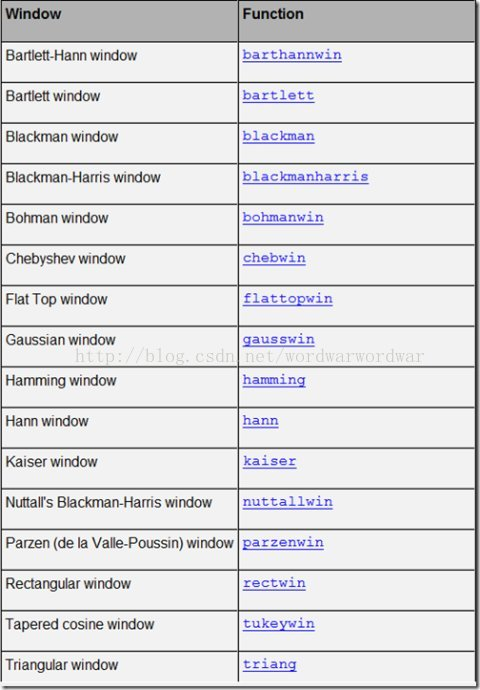​ 要确定如何设计一个FIR滤波器，首先得对加窗后的理想滤波器特性变化进行分析，并研究减少由截断引起的误差的途径，从而提出更好的滤波器设计方案。对于调整窗口长度可以有效地控制过渡带的宽度，但减少带内波动以及加大阻带衰减没有作用。所以必须挑选最为合适的窗函数对理想滤波器进行截取。下面简单介绍几种窗函数。一个实际的滤波器的单位脉冲响应可表示为：

h

(

n

)

=

h

d

(

n

)

∗

w

(

n

)

h(n) = h_d(n)*w(n)

几种常见窗函数（只给出了窗函数的定义和幅度特性）：

W

(

e

j

∗

w

)

=

W

(

w

)

(

e

−

j

α

w

)

W(e^{j*w})=W(w)(e^{-jαw})

矩形窗FIR滤波器设计:
矩形窗的窗函数为：

w

R

(

n

)

=

R

N

(

n

)

w_R(n)=R_N(n)

幅度函数为：

R

N

(

w

)

=

s

i

n

(

w

N

/

2

)

s

i

n

(

w

/

2

)

R_N(w) = \frac{sin(wN/2)}{sin(w/2)}

它的主瓣宽度为

4

π

/

N

4\pi/N

,第一瓣比主瓣地13dB.
在Matlab中，实现矩形窗的函数为boxcar和recttwin ,其调用格式如下：
w=boxcar(N);
w=recttwin(N);
%%%显示窗函数的GUI工具
n = 60;
w = rectwin(n);
wvtool(w)%显示窗函数的GUI工具
%还提供了显示窗函数的GUI工具，如wvtool可以显示用来显示窗的形状和频域图形，wintool可以打开窗设计和分析工具，如运行
wvtool(hamming(64),hann(64),gausswin(64))
%%可以对比汉明窗、汉宁窗和高斯窗

其中，N是窗函数的长度，返回值w是一个N阶的向量，它的元素有窗函数的值组成。其中w=boxcar(N)等价于w=ones(N,1)。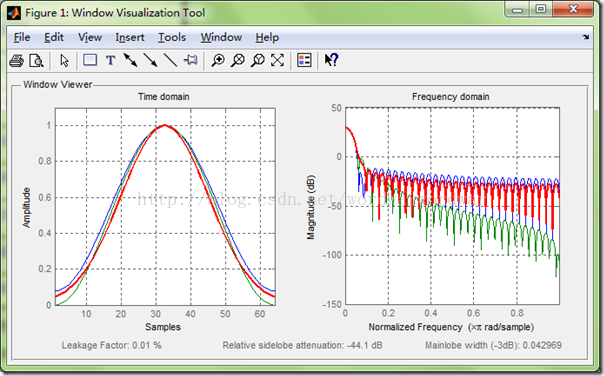案例分析：
利用矩形窗设计FIR带阻滤波器，主要参数如下:
给定抽样频率为

Ω

s

=

2

∗

p

i

∗

1.5

∗

1

0

4

(

r

a

d

/

s

e

c

)

,
通带截至频率为

Ω

p

1

=

2

∗

p

i

∗

0.75

∗

1

0

3

(

r

a

d

/

s

e

c

)

,

Ω

p

2

=

2

∗

p

i

∗

6

∗

1

0

3

(

r

a

d

/

s

e

c

)

阻带截至频率为

Ω

s

t

1

=

2

∗

p

i

∗

2.25

∗

1

0

3

(

r

a

d

/

s

e

c

)

,

Ω

s

t

2

=

2

∗

p

i

∗

1.5

∗

1

0

3

(

r

a

d

/

s

e

c

)

阻带衰减

δ

2

>

=

50

d

B

{\delta}_2 >=50dB

。
%%%%调用子程序1：
function hd=ideal_bs(Wcl,Wch,m);
alpha=(m-1)/2;
n=[0:1:(m-1)];
m=n-alpha+eps;
hd=[sin(m*pi)+sin(Wcl*m)-sin(Wch*m)]./(pi*m)
%%%%调用子程序2：
function[db,mag,pha,w]=freqz_m2(b,a)
[H,w]=freqz(b,a,1000,'whole');
H=(H(1:1:501))'; w=(w(1:1:501))';
mag=abs(H);
db=20*log10((mag+eps)/max(mag));
pha=angle(H);
%%%%运行MATLAB源代码如下：
clear all;
Wph=3*pi*6.25/15;%通带频率
Wpl=3*pi/15;
Wsl=3*pi*2.5/15;%阻带频率
Wsh=3*pi*4.75/15;
tr_width=min((Wsl-Wpl),(Wph-Wsh));%%过渡带带宽
%过渡带宽度
N=ceil(4*pi/tr_width);					%滤波器长度
n=0:1:N-1;
Wcl=(Wsl+Wpl)/2;						%理想滤波器的截止频率
Wch=(Wsh+Wph)/2;
hd=ideal_bs(Wcl,Wch,N);				%理想滤波器的单位冲击响应
w_ham=(boxcar(N))';
string=['矩形窗','N=',num2str(N)];
h=hd.*w_ham;						%截取取得实际的单位脉冲响应
[db,mag,pha,w]=freqz_m2(h,);
%计算实际滤波器的幅度响应
delta_w=2*pi/1000;
subplot(241);
stem(n,hd);
title('理想脉冲响应hd(n)')
axis([-1,N,-0.5,0.8]);
xlabel('n');ylabel('hd(n)');
grid on
subplot(242);
stem(n,w_ham);
axis([-1,N,0,1.1]);
xlabel('n');ylabel('w(n)');
text(1.5,1.3,string);
grid on
subplot(243);
stem(n,h);title('实际脉冲响应h(n)');
axis([0,N,-1.4,1.4]);
xlabel('n');ylabel('h(n)');
grid on
subplot(244);
plot(w,pha);title('相频特性');
axis([0,3.15,-4,4]);
grid on
subplot(245);
plot(w/pi,db);title('幅度特性（dB）');
axis([0,1,-80,10]);
xlabel('频率（pi）');ylabel('分贝数');
grid on
subplot(246);
plot(w,mag);title('频率特性')
axis([0,3,0,2]);
grid on
fs=15000;
t=(0:100)/fs;
x=cos(2*pi*t*750)+cos(2*pi*t*3000)+cos(2*pi*t*6100);
q=filter(h,1,x);
[a,f1]=freqz(x);
f1=f1/pi*fs/2;
[b,f2]=freqz(q);
f2=f2/pi*fs/2;
subplot(247);
plot(f1,abs(a));
title('输入波形频谱图');
xlabel('频率');ylabel('幅度')
grid on
subplot(248);
plot(f2,abs(b));
title('输出波形频谱图');
xlabel('频率');ylabel('幅度')
grid on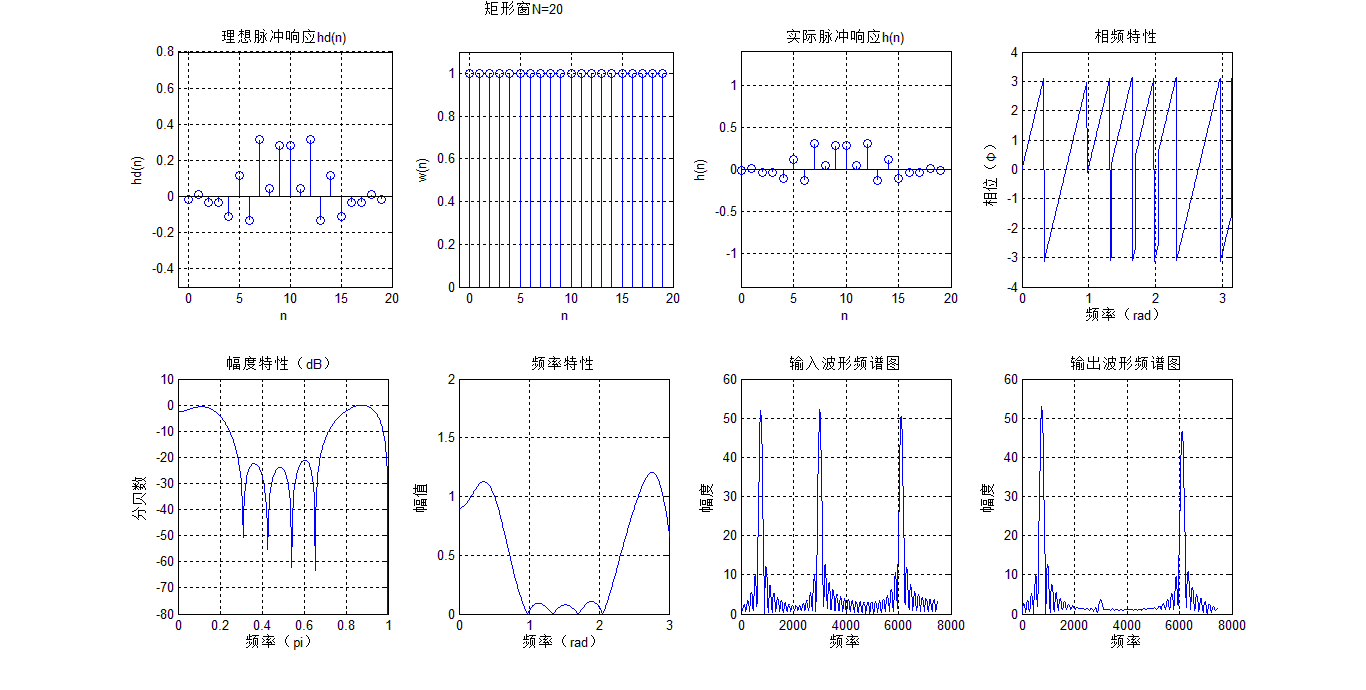汉宁窗FIR滤波器设计:
汉宁窗（hanning window）又称升余弦窗，窗函数为：

w

H

n

(

n

)

=

0.5

[

1

−

c

o

s

(

2

π

∗

n

N

−

1

)

]

∗

R

N

(

n

)

w_{Hn}(n) = 0.5[1-cos(\frac{2\pi*n}{N-1})]*R_N(n)

幅值函数为：

W

H

n

(

w

)

=

0.5

W

R

(

w

)

+

0.25

[

W

R

(

w

−

2

π

N

−

1

)

+

W

R

(

w

+

2

π

N

−

1

)

]

W_{Hn}(w) = 0.5W_R(w) + 0.25[W_R(w-\frac{2\pi}{N-1})+W_R(w+\frac{2\pi}{N-1})]

汉宁窗幅度函数由3部分相加而成，其结果是使主瓣集中了更多能量，而旁瓣3部分相加时相互抵消而变小，其代价是主瓣宽度增加到

8

π

/

N

8\pi/N

。第一瓣比主瓣低31dB,阻带衰减加大。
在Matlab中，实现汉宁窗的函数为hanning和barthannwin ,其调用格式如下：
w=hanning(N)
w=barthannwin(N)

案例1：绘制50个点的汉宁窗。
N=49;n=1:N;
wdhn=hanning(N);
figure(3);
stem(n,wdhn,'.');
grid on
axis([0,N,0,1.1]);
title('50点汉宁窗');
ylabel('W(n)');
xlabel('n');
title('50点汉宁窗');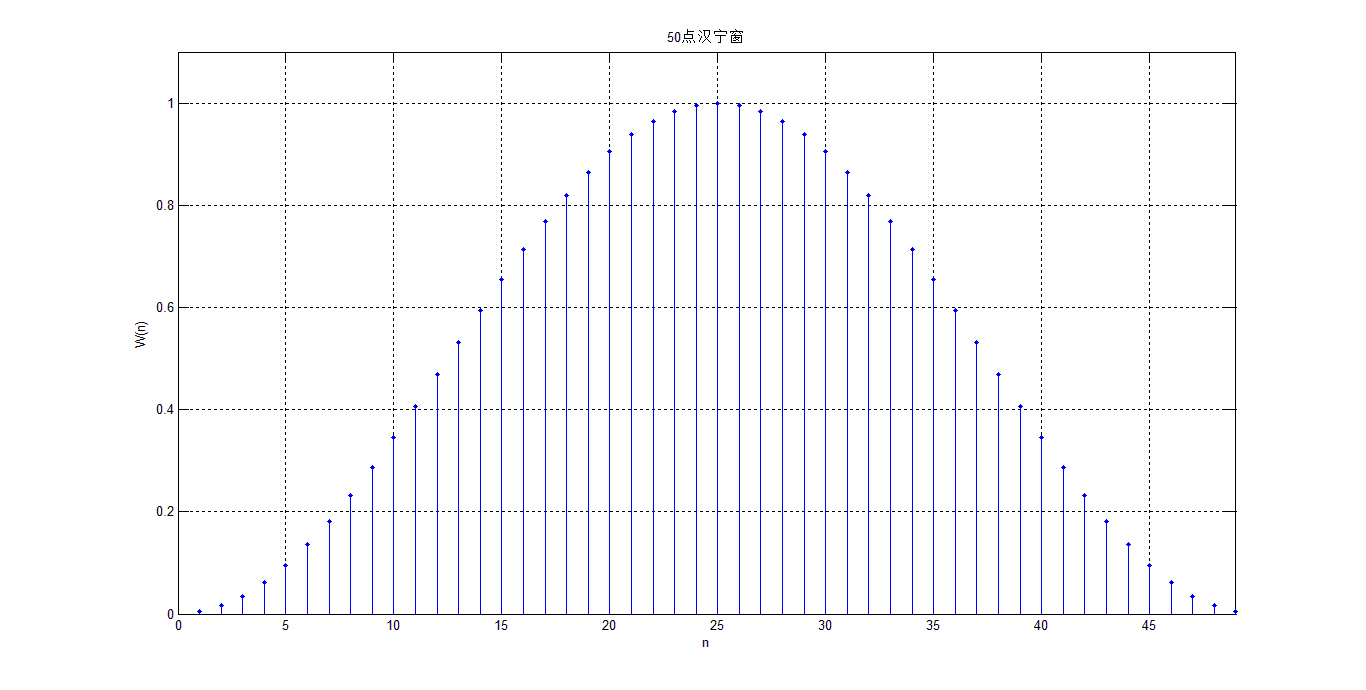案例2：已知连续信号为

x

(

t

)

=

c

o

s

(

2

π

f

1

t

)

+

0.15

c

o

s

(

2

π

f

2

t

)

x(t)=cos(2{\pi}f_1t) +0.15cos(2{\pi}f_2t)

，其中

f

1

=

100

H

z

,

f

2

=

150

H

z

f_1=100Hz,f_2=150Hz

。若抽样频率

f

s

a

m

=

600

H

z

f_sam=600Hz

d对信号进行抽样，利用不同宽度N的矩形截断该序列，N取40，观察不同的窗对普分析结果的影响。
N=40;
L=512;
f1=100;f2=150;fs=600;
ws=2*pi*fs;
t=(0:N-1)*(1/fs);
x=cos(2*pi*f1*t)+0.25*sin (2*pi*f2*t);
wh=boxcar(N)';
x=x.*wh;
subplot(221);stem(t,x);
title('加矩形窗时域图');
xlabel('n');ylabel('h(n)')
grid on
W=fft(x,L);
f=((-L/2:L/2-1)*(2*pi/L)*fs)/(2*pi);
subplot(222);
plot(f,abs(fftshift(W)))
title('加矩形窗频域图');
xlabel('频率');ylabel('幅度')
grid on
x=cos(2*pi*f1*t)+0.15*cos(2*pi*f2*t);
wh=hanning(N)';
x=x.*wh;
subplot(223);stem(t,x);
title('加汉宁窗时域图');
xlabel('n');ylabel('h(n)')
grid on
W=fft(x,L);
f=((-L/2:L/2-1)*(2*pi/L)*fs)/(2*pi);
subplot(224);
plot(f,abs(fftshift(W)))
title('加汉宁窗频域图');
xlabel('频率');ylabel('幅度')
grid on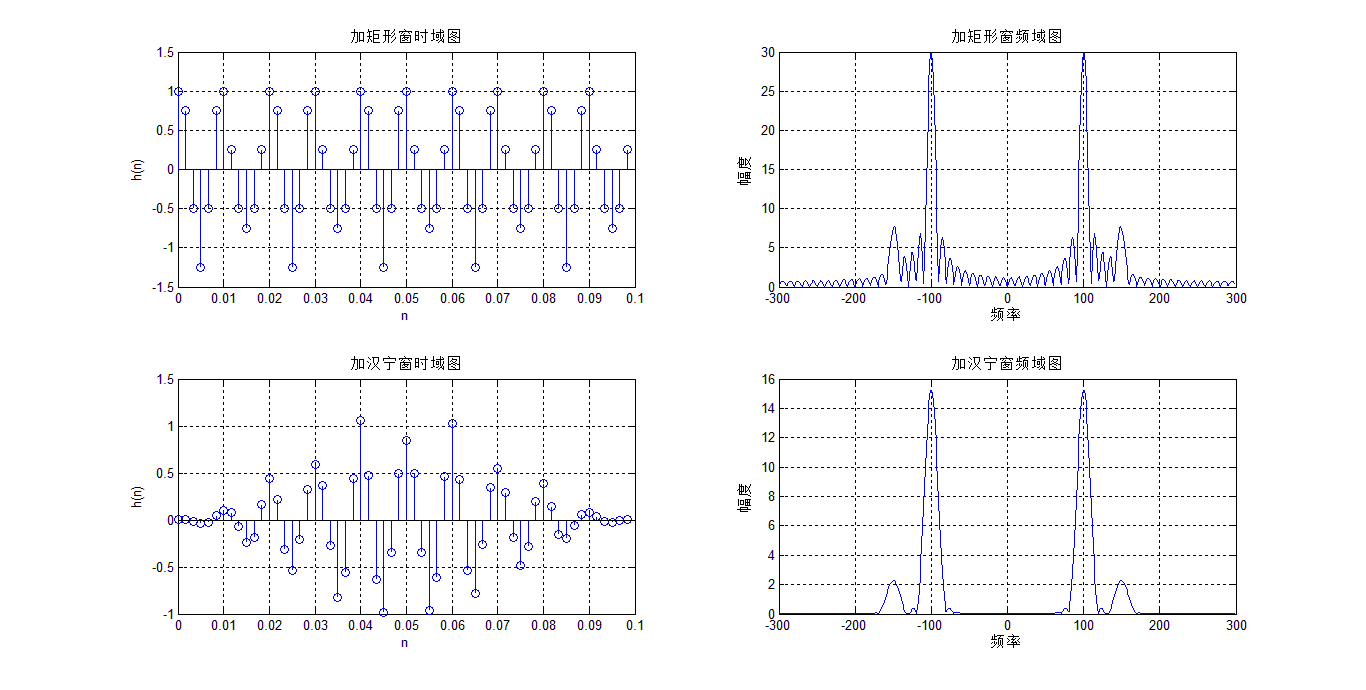用汉宁窗对谐波信号进行分析：
clear;
% 原始数据：直流:0V; 基波：49.5Hz,100V,10deg; HR2:0.5V,40deg;
hr0=0;f1=50.1;
hr(1)=25*sqrt(2);deg(1)=10;
hr(2)=0;deg(2)=0;
hr(3)=1.755*sqrt(2);deg(3)=40;
hr(4)=0;deg(4)=0;
hr(5)=0.885*sqrt(2);deg(5)=70;
hr(6)=0;deg(6)=0;
hr(7)=1.125;deg(7)=110;
M=7;f=[1:M]*f1;							%设定频率
% 采样
fs=10000;
N=2048;									% 约10个周期
T=1/fs;
n=[0:N-1];t=n*T;
x=zeros(size(t));
for k=1:M
x=x+hr(k)*cos(2*pi*f(k)*t+deg(k)*pi/180);
end
%分析：
w=0.5-0.5*cos(2*pi*n/N);
Xk=fft(x.*w);
amp=abs(Xk(1:N/2))/N*2;						%幅频
pha=angle(Xk(1:N/2))/pi*180;					%相频
for k=1:N/2
if(amp(k)<0.01) pha(k)=0;  %当谐波<10mV时，其相位=0
end
if(pha(k)<0) pha(k)=pha(k)+360;%调整到0-360度
end
end
fmin=fs/N;
xaxis=fmin*n(1:N/2);
%横坐标为Hz
kx=round([1:M]*50/fmin);
%各次谐波对应的下标(从0开始)
for m=1:M
km(m)=searchpeaks(amp,kx(m)+1);			%km为谱峰(从1开始)
if(amp(km(m)+1)<amp(km(m)-1))
km(m)=km(m)-1;
end
beta(m)=amp(km(m)+1)./amp(km(m));
delta(m)=(2*beta(m)-1)./(1+beta(m));
end
fx=(km-1+delta)*fmin;							%估计频率
hrx=amp(km)*2.*pi.*delta.*(1-delta.*delta)./sin(pi*delta);
degx=pha(km)-delta.*180/N*(N-1);				%估计相位
degx=mod(degx,360);							%调整到0-360度
efx=(fx-f)./f*100;								%频率误差
ehr=(hrx-hr)./hr*100;							%幅度误差
edeg=(degx-deg);								%相位误差
% 结果输出：
subplot(2,2,1);
%画出采样序列
plot(t,x);
hold on;
plot(t,x.*w,'r');
%加窗波形
hold off;
xlabel('x(k)');
title('原信号和加窗信号 ');
subplot(2,2,2);
%画出FFT分析结果
stem(xaxis,amp,'.r');
xlabel('频率');
title('幅频结果');
subplot(2,2,4);
stem(xaxis,pha,'.r');
xlabel('角频率');
title('相频结果');
subplot(2,2,3);
stem(ehr);
title('幅度误差(%)');
%文本输出
fid=fopen('result.txt','w');
fprintf(fid,'原始数据：f1=%6.1fHz, N=%.f,  fs=%.f \r\n\r\n',f1,N,fs);
fprintf(fid,'谐波次数      1      2      3      4      5      6     7\r\n');
fprintf(fid,'设定频率 %6.3f %6.3f %6.3f %6.3f %6.3f %6.3f %6.3f\r\n',f);
fprintf(fid,'估计频率 %6.3f %6.3f %6.3f %6.3f %6.3f %6.3f %6.3f\r\n',fx);
fprintf(fid,'误差(%%)  %6.3f %6.3f %6.3f %6.3f %6.3f %6.3f %6.3f\r\n\r\n',efx);
fprintf(fid,'设定幅值 %6.3f %6.3f %6.3f %6.3f %6.3f %6.3f %6.3f\r\n',hr);
fprintf(fid,'估计幅值 %6.3f %6.3f %6.3f %6.3f %6.3f %6.3f %6.3f\r\n',hrx);
fprintf(fid,'误差(%%)  %6.3f %6.3f %6.3f %6.3f %6.3f %6.3f %6.3f\r\n\r\n',ehr);
fprintf(fid,'设定相位 %6.2f %6.2f %6.2f %6.2f %6.2f %6.2f %6.2f\r\n',deg);
fprintf(fid,'估计相位 %6.2f %6.2f %6.2f %6.2f %6.2f %6.2f %6.2f\r\n',degx);
fprintf(fid,'误差(度)  %6.2f %6.2f %6.2f %6.2f %6.2f %6.2f %6.2f\r\n\r\n',edeg);
%其他数据
fprintf(fid,'谱峰位置理论值：\r\n %6.4f %6.4f %6.4f %6.4f %6.4f %6.4f %6.4f\r\n',[1:M]*f1/fmin);
fprintf(fid,'谱峰位置估计值：\r\n %6.4f %6.4f %6.4f %6.4f %6.4f %6.4f %6.4f\r\n',km-1+delta);
fprintf(fid,'误差（%%）\r\n %6.4f %6.4f %6.4f %6.4f %6.4f %6.4f %6.4f\r\n',((km-1+delta)-[1:M]*f1/fmin)./([1:M]*f1/fmin)*100);
fprintf(fid,'delta     ：\r\n %6.4f %6.4f %6.4f %6.4f %6.4f %6.4f %6.4f\r\n',delta);
fclose(fid);

%运行过程中的调用子程序：
function index1=searchpeaks(x,index)
%在数组中寻找最大值对应的下标
%x为数组，index 为给定的下标(index不能取最前或最后2个下标)，在前后2个数中（共5个数）查找最大值和紧邻的次最大值
% indexmax 返回两个谱峰位置中的前一个谱峰对应的下标
index1=index-2;
for k=-1:2
if(x(index+k)>x(index1))
index1=index+k;
end
end
if x(index1-1)>x(index1+1)
index1=index1-1;
end


#result.txt输出结果
原始数据：f1=  50.1Hz, N=2048,  fs=10000

谐波次数      1      2      3      4      5      6     7
设定频率 50.100 100.200 150.300 200.400 250.500 300.600 350.700
估计频率 50.100 78.819 150.302 181.252 250.499 279.138 350.701
误差(%)  -0.000 -21.338  0.001 -9.555 -0.000 -7.140  0.000

设定幅值 35.355  0.000  2.482  0.000  1.252  0.000  1.125
估计幅值 35.356  0.046  2.482  0.002  1.252  0.002  1.125
误差(%)   0.001    Inf  0.009    Inf  0.004    Inf  0.003

设定相位  10.00   0.00  40.00   0.00  70.00   0.00 110.00
估计相位  10.03  31.67  39.97 338.35  70.06 329.86 110.05
误差(度)    0.03  31.67  -0.03 338.35   0.06 329.86   0.05

谱峰位置理论值：
10.2605 20.5210 30.7814 41.0419 51.3024 61.5629 71.8234
谱峰位置估计值：
10.2605 16.1421 30.7818 37.1203 51.3022 57.1675 71.8235
误差（%）
-0.0002 -21.3385 0.0012 -9.5551 -0.0004 -7.1396 0.0002
delta     ：
0.2605 0.1421 0.7818 0.1203 0.3022 0.1675 0.8235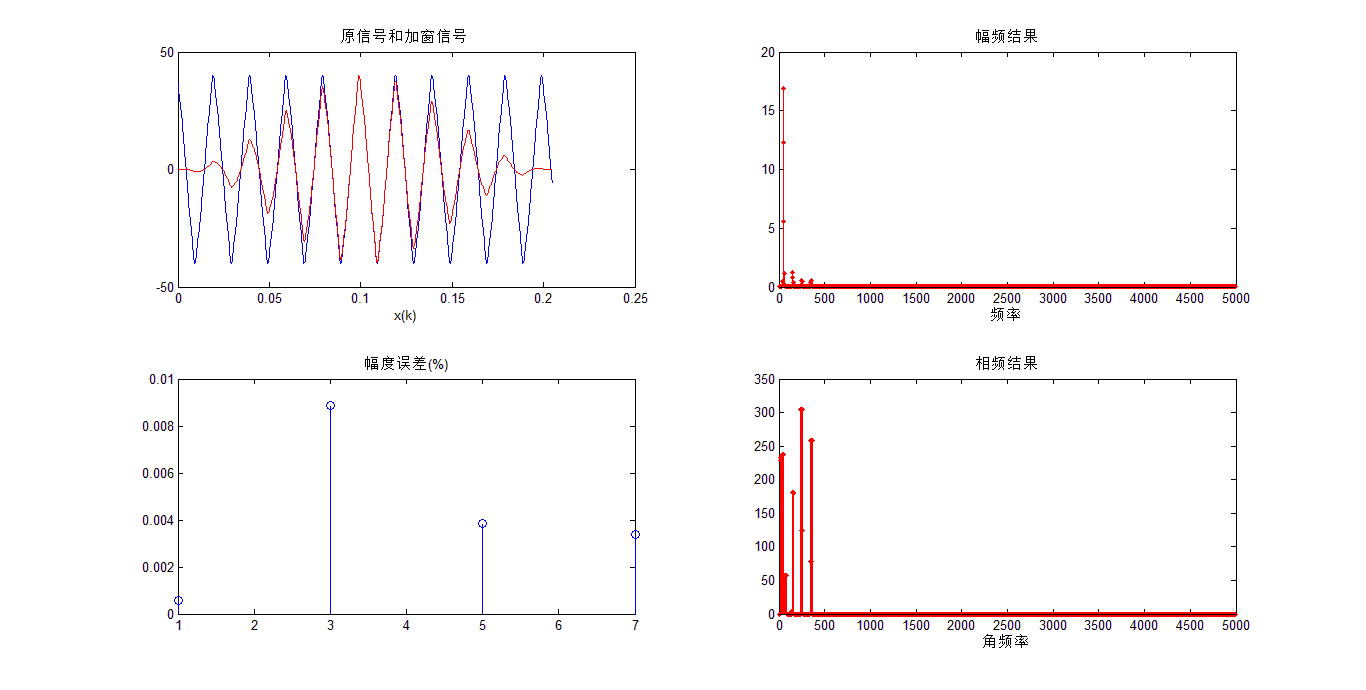汉明窗FIR滤波器设计:
汉宁窗（hanming window）又称改进升余弦窗，窗函数为：

w

H

n

(

n

)

=

[

0.54

−

0.46

∗

c

o

s

(

2

π

∗

n

N

−

1

)

]

∗

R

N

(

n

)

w_{Hn}(n) = [0.54-0.46*cos(\frac{2\pi*n}{N-1})]*R_N(n)

幅值函数为：

W

H

n

(

w

)

=

0.54

W

R

(

w

)

+

0.23

[

W

R

(

w

−

2

π

N

−

1

)

+

0.23

W

R

(

w

+

2

π

N

−

1

)

]

W_{Hn}(w) = 0.54W_R(w) + 0.23[W_R(w-\frac{2\pi}{N-1})+0.23W_R(w+\frac{2\pi}{N-1})]

汉明窗主瓣宽度与汉宁窗相同，

8

π

/

N

，

99.96

%

8{\pi}/N，99.96\%

的能量集中在主瓣，第一瓣比主瓣低41dB。
在Matlab中，实现汉宁窗的函数为hanming ,其调用格式如下：
w=hanming(N)

案例分析1：设计一个汉明窗低通滤波器：
%语音信号设计一个汉明窗低通滤波器：
x=x(:,1);
figure(1);
subplot(211);plot(x);
title('语音信号时域波形图')
xlabel('n');ylabel('h(n)')
grid on
y=fft(x,1000);
f=(FS/1000)*[1:1000];
subplot(212);
plot(f(1:300),abs(y(1:300)));
title('语音信号频谱图');
xlabel('频率');ylabel('幅度')
grid on
%产生噪声信号并加到语音信号
t=0:length(x)-1;
zs0=0.05*cos(2*pi*10000*t/1024);
zs=[zeros(0,20000),zs0];
figure(2);
subplot(211)
plot(zs)
title('噪声信号波形');
xlabel('n');ylabel('h(n)')
grid on
zs1=fft(zs,1200);
subplot(212)
plot(f(1:600),abs(zs1(1:600)));
title('噪声信号频谱');
xlabel('频率');ylabel('幅度')
grid on
x1=x+zs';
%sound(x1,FS,bits);
y1=fft(x1,1200);
figure(3);
subplot(211);plot(x1);
title('加入噪声后的信号波形');
xlabel('n');ylabel('h(n)')
grid on
subplot(212);
plot(f(1:600),abs(y1(1:600)));
title('加入噪声后的信号频谱');
xlabel('频率');ylabel('幅度')
grid on
%滤波
fp=7500;
fc=8500;
wp=2*pi*fp/FS;
ws=2*pi*fc/FS;
Bt=ws-wp;
N0=ceil(6.2*pi/Bt);
N=N0+mod(N0+1,2);
wc=(wp+ws)/2/pi;
hn=fir1(N-1,wc,hamming(N));
X=conv(hn,x);
X1=fft(X,1200);
figure(4);
subplot(211);
plot(X);
title('滤波后的信号波形');
xlabel('n');ylabel('h(n)')
grid on
subplot(212);
plot(f(1:600),abs(X1(1:600)));
title('滤波后的信号频谱')
xlabel('频率');ylabel('幅度')
grid on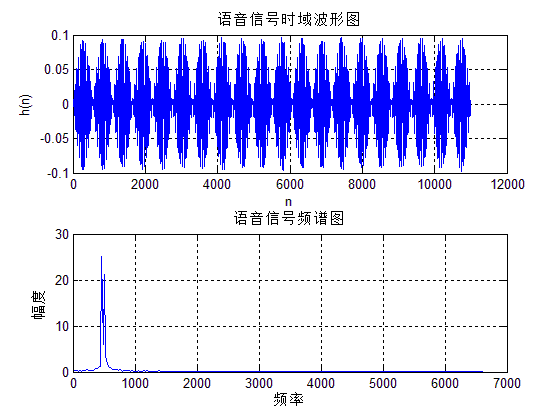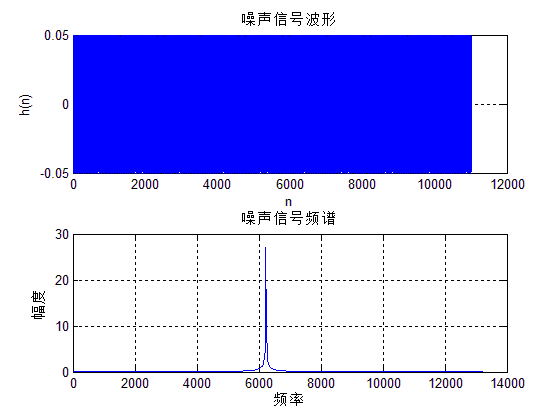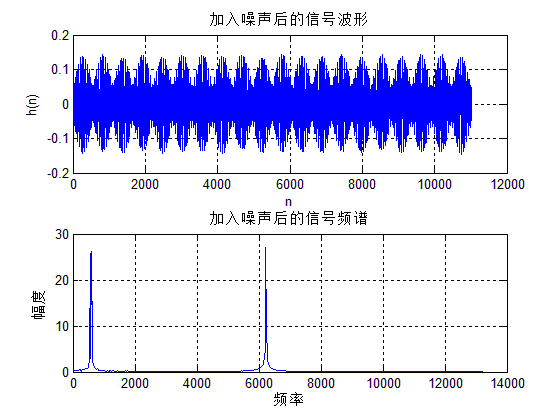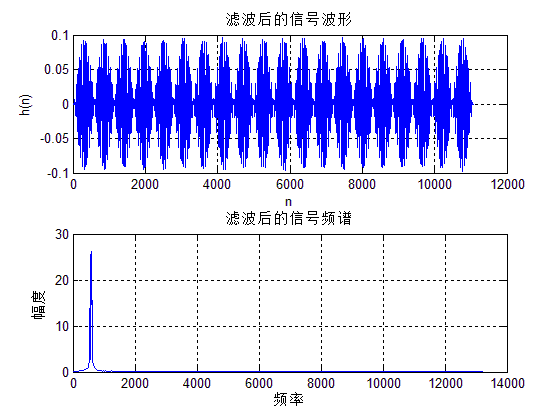案例分析2：已知连续信号为

x

a

(

t

)

=

c

o

s

(

100

π

t

)

+

s

i

n

(

100

π

t

)

+

c

o

s

(

50

π

t

)

x_a(t)=cos(100{\pi}t) +sin(100{\pi}t)+cos(50{\pi}t)

，用DFT分析其中

x

a

(

t

)

x_a(t)

的频谱结构，选择不同的截取长度

T

p

T_p

。观察存在的截断效应，试用加窗的方法减少谱间干扰。
clear;close all
fs=400;T=1/fs;						%采样频率和采样间隔
Tp=0.04;N=Tp*fs;					%采样点数N
N1=[N,4*N,8*N];					%设定三种截取长度
for m=1:3
n=1:N1(m);
xn=cos(100*pi*n*T)+ sin(200*pi*n*T)+ cos(50*pi*n*T);
Xk=fft(xn,4096);
fk=[0:4095]/4096/T;
subplot(3,2,2*m-1);plot(fk,abs(Xk)/max(abs(Xk)));
if m==1 title('矩形窗截取');
end
end
%hamming窗截断
for m=1:3
n=1:N1(m);
wn=hamming(N1(m));
xn=cos(200*pi*n*T)+ sin(100*pi*n*T)+ cos(50*pi*n*T).*wn';
Xk=fft(xn,4096);
fk=[0:4095]/4096/T;
subplot(3,2,2*m);plot(fk,abs(Xk)/max(abs(Xk)));
if m==1 title('hamming窗截取');
end
end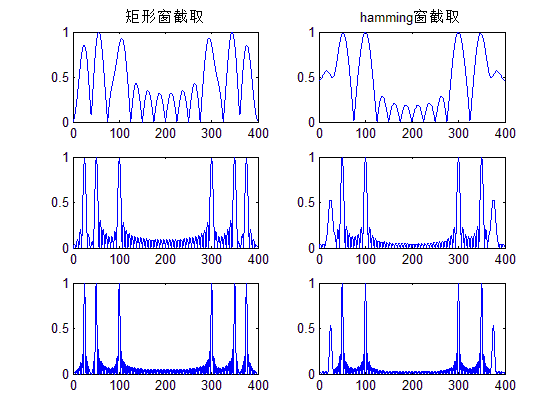比较矩形窗与汉明窗的普分析结果可见，矩形窗比用汉明窗分辨率高（泄露小），但是谱间干扰大。因此汉明窗是以牺牲分辨率来换取谱间干扰降低。
布莱克曼FIR滤波器设计:
布莱克曼（Blackman window）的窗函数为：

w

B

l

(

n

)

=

[

0.42

−

0.5

∗

c

o

s

(

2

π

∗

n

N

−

1

)

+

0.08

∗

c

o

s

(

4

π

∗

n

N

−

1

)

]

∗

R

N

(

n

)

w_{Bl}(n) = [0.42-0.5*cos(\frac{2\pi*n}{N-1})+0.08*cos(\frac{4\pi*n}{N-1})]*R_N(n)

幅值函数为：

W

H

n

(

w

)

=

0.42

W

R

(

w

)

+

0.25

[

W

R

(

w

−

2

π

N

−

1

)

+

W

R

(

w

+

2

π

N

−

1

)

]

W_{Hn}(w) = 0.42W_R(w) + 0.25[W_R(w-\frac{2\pi}{N-1})+W_R(w+\frac{2\pi}{N-1})]

+

0.04

[

W

R

(

w

−

4

π

N

−

1

+

W

R

(

w

+

4

π

N

−

1

)

]

+0.04[W_R(w-\frac{4\pi}{N-1}+W_R(w+\frac{4\pi}{N-1})]

布莱克曼窗幅度函数由5部分相加而成，5部分相加的结果使得旁瓣得到进一步抵消，阻带衰减加大而过渡带加大到

12

π

/

N

12{\pi}/N

。
在Matlab中，实现布莱克曼窗的函数为blackman ,其调用格式如下：
w=blackman(N);

:案例：用窗函数法设计数字带通滤波器。下阻带边缘：

W

s

1

=

0.3

π

，

A

s

=

65

d

B

W_{s1}=0.3{\pi}，A_s=65dB

，下通带边缘:

W

p

1

=

0.4

π

，

R

p

=

1

d

B

W_{p1}=0.4{\pi}，R_p=1dB

,上通带边缘：

W

p

2

=

0.6

π

，

R

p

=

1

d

B

W_{p2}=0.6{\pi}，R_p=1dB

，上阻带边缘:

W

s

2

=

0.7

π

，

R

p

=

65

d

B

W_{s2}=0.7{\pi}，R_p=65dB

。根据窗函数最小阻带衰减的特性，以及参照窗函数的基本参数表，选择布莱克曼窗可以达到75dB的最小阻带衰减，其过渡带为

11

π

/

N

11\pi/N

。
clear all;
wp1=0.4*pi;
wp2=0.6*pi;
ws1=0.3*pi;
ws2=0.7*pi;
As=65;
tr_width=min((wp1-ws1),(ws2-wp2)); 					%过渡带宽度
M=ceil(11*pi/tr_width)+1							%滤波器长度
n=[0:1:M-1];
wc1=(ws1+wp1)/2;									%理想带通滤波器的下截止频率
wc2=(ws2+wp2)/2;									%理想带通滤波器的上截止频率
hd=ideal_lp(wc2,M)-ideal_lp(wc1,M);
w_bla=(blackman(M))';								%布莱克曼窗
h=hd.*w_bla;
%截取得到实际的单位脉冲响应
[db,mag,pha,grd,w]=freqz_m(h,);
%计算实际滤波器的幅度响应
delta_w=2*pi/1000;
Rp=-min(db(wp1/delta_w+1:1:wp2/delta_w))
%实际通带纹波
As=-round(max(db(ws2/delta_w+1:1:501)))
As=75
subplot(2,2,1);
stem(n,hd);
title('理想单位脉冲响应hd(n)')
axis([0 M-1 -0.4 0.5]);
xlabel('n');
ylabel('hd(n)')
grid on;
subplot(2,2,2);
stem(n,w_bla);
title('布莱克曼窗w(n)')
axis([0 M-1 0 1.1]);
xlabel('n');
ylabel('w(n)')
grid on;
subplot(2,2,3);
stem(n,h);
title('实际单位脉冲响应hd(n)')
axis([0 M-1 -0.4 0.5]);
xlabel('n');
ylabel('h(n)')
grid on;
subplot(2,2,4);
plot(w/pi,db);
axis([0 1 -150 10]);
title('幅度响应(dB)');
grid on;
xlabel('频率单位:pi');
ylabel('分贝数')

%调用小程序设计1：
function [db,mag,pha,grd,w] = freqz_m(b,a);
% Modified version of freqz subroutine
% ------------------------------------
% [db,mag,pha,grd,w] = freqz_m(b,a);
%  db = Relative magnitude in dB computed over 0 to pi radians
% mag = absolute magnitude computed over 0 to pi radians
% pha = Phase response in radians over 0 to pi radians
% grd = Group delay over 0 to pi radians
%   w = 501 frequency samples between 0 to pi radians
%   b = numerator polynomial of H(z)   (for FIR: b=h)
%   a = denominator polynomial of H(z) (for FIR: a=)
%
[H,w] = freqz(b,a,1000,'whole');
H = (H(1:1:501))'; w = (w(1:1:501))';
mag = abs(H);
db = 20*log10((mag+eps)/max(mag));
pha = angle(H);
%  pha = unwrap(angle(H));
grd = grpdelay(b,a,w);
%  grd = diff(pha);
%  grd = [grd(1) grd];
%  grd = [0 grd(1:1:500); grd; grd(2:1:501) 0];
%  grd = median(grd)*500/pi;

%调用小程序设计2：
function hd=ideal_lp(wc,M);
%计算理想低通滤波器的脉冲响应
%[hd]=ideal_lp(wc,M)
%hd=理想脉冲响应0到M-1
%wc=截止频率
% M=理想滤波器的长度
alpha=(M-1)/2;
n=[0:1:(M-1)];
m=n-alpha+eps;
%加上一个很小的值eps避免除以0的错误情况出现
hd=sin(wc*m)./(pi*m);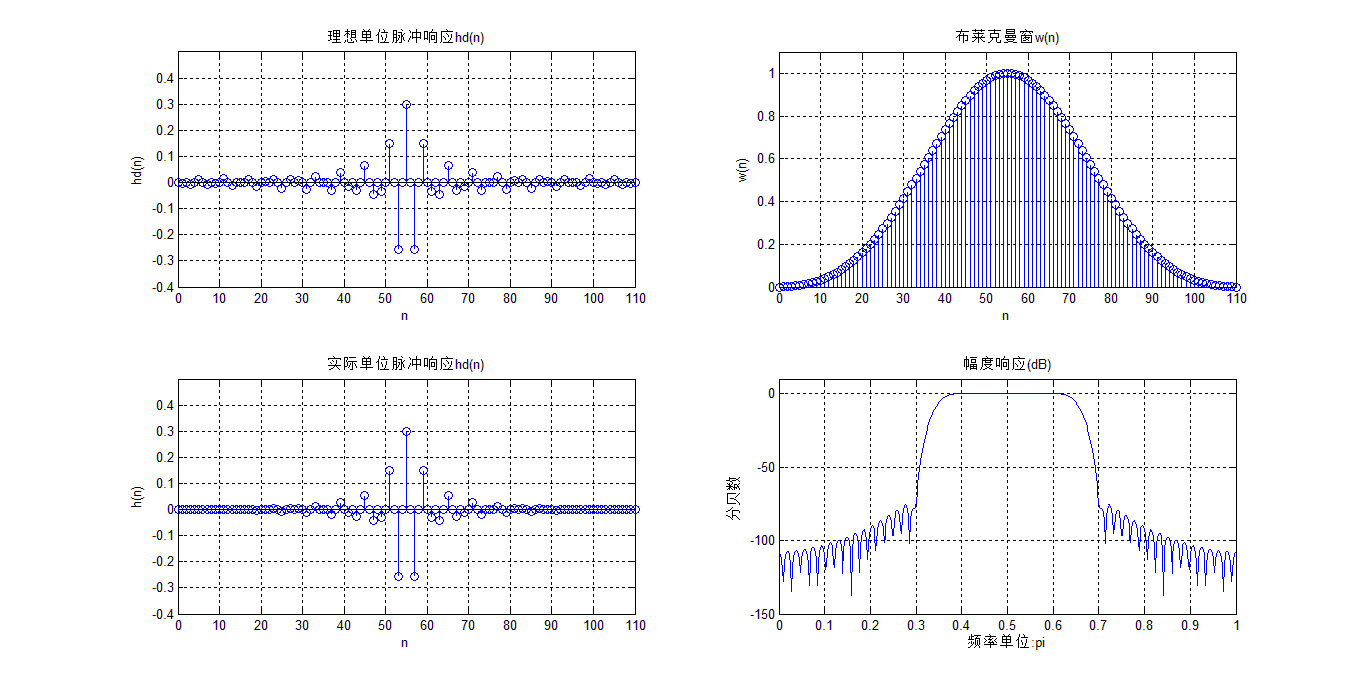凯塞窗FIR滤波器设计:
凯塞-贝塞窗（Kaiser-Basel window)的窗函数为：

w

k

(

n

)

=

I

0

(

β

)

I

0

(

α

)

∗

R

N

(

n

)

w_{k}(n) = \frac{I_0( β )}{I_0( \alpha )}*R_N(n)

式中，

β

=

α

(

1

−

(

2

n

N

−

1

)

−

1

)

2

\beta= {\alpha}{\sqrt{(1-(\frac{2n}{N-1})-1)^2}}

。

I

0

(

x

)

I_0( x )

是零阶第一类修正贝塞函数，可用下面级数计算：

I

0

(

x

)

=

1

+

∑

k

=

1

+

∞

（

1

k

!

(

x

2

)

k

）

2

I_0( x ) = 1+\sum_{k=1}^{+ ∞}（\frac{1}{k!}({\frac{x}{2}})^{k}）^{2}

​

I

0

(

x

)

I_0( x )

q取15-25项就可以满足精度要求。通常

α

\alpha

用以控制窗的形状，

α

\alpha

加大，主瓣加宽，旁瓣减小，典型数据

4

<

α

<

9

4<\alpha<9

。当

α

=

5.44

\alpha=5.44

s时，窗函数接近汉明窗；当

α

=

7.865

\alpha=7.865

s时，窗函数接近于布莱克曼窗。其幅值函数为：

W

k

(

w

)

=

w

k

(

0

)

+

2

∑

n

=

1

(

N

−

1

)

2

(

w

k

(

n

)

c

o

s

(

w

n

)

)

W_{k}(w) =w_k(0) + 2\sum_{n=1}^{\frac{(N-1)}{2}}(w_k(n)cos(wn))

在Matlab中，实现汉宁窗的函数为kaiser,其调用格式如下：
w=kaiser(N);
在Matlab中设计标准响应FIR滤波器可使用fir1函数。fir1函数以经典方法实现加窗性相位FIR滤波器的设计，它可以设计出标准的低通、高通、带通、带阻滤波器。fir1函数用法为：
b = fir1(n,Wn,‘ftype’,wimdow)
各个参数的含义如下：
b -滤波器系数，n-滤波器阶数。Wn -截止频率，

0

<

=

W

n

<

=

1

0<=W_n<=1

,

W

n

=

1

W_n=1

对应于采样频率的一半。当设计带通和带阻滤波器时，

W

n

=

[

W

1

,

W

2

]

,

W

1

<

w

<

W

2

W_n =[W_1,W_2],W_1<w<W_2

ftype -当指定ftype时，可设计高通和带阻滤波器。ftype=hight时，设计高通FIR滤波器；ftype=stop时设计带阻FIR滤波器。低通和带通FIR滤波器无需输入ftype参数。window–窗函数。窗函数的长度应等于FIR滤波器系数的个数，即阶数n+1。
案例分析：利用凯塞窗函数设计一个带通滤波器，上截止频率2500Hz,下截止频率1000Hz，过渡带宽200Hz,通带纹波允许差0.1，带阻纹波不大于允差0.02dB，通带幅值为1。
Fs=8000;N=216;
fcuts=[1000 1200 2300 2500];
mags=[0 1 0];
devs=[0.02 0.1 0.02];
[n,Wn,beta,ftype]=kaiserord(fcuts,mags,devs,Fs);
n=n+rem(n,2);
hh=fir1(n,Wn,ftype,kaiser(n+1,beta),'noscale');
[H,f]=freqz(hh,1,N,Fs);
plot(f,abs(H));
xlabel('频率 (Hz)');
ylabel('幅值|H(f)|');
grid on;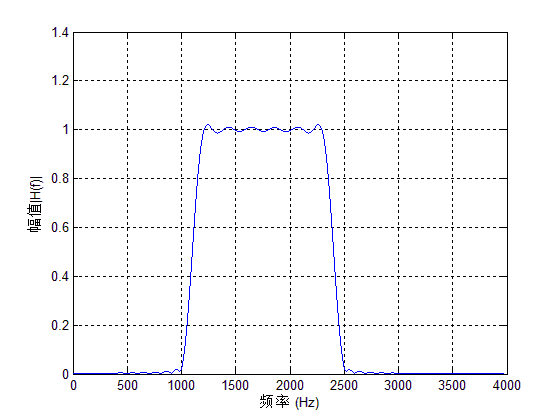窗函数设计法：
根据前面几节的分析：设计一个FIR低通滤波器通常按照下面的步骤执行：
根据滤波器设计要求，确定滤波器的过渡带宽和阻带衰减要求，选择合适窗函数的类型并进行估计窗函数的宽度N。根据所求的理想滤波器求出单位脉冲响应

h

d

(

n

)

h_d(n)

根据求得的h(n)求出其频率响应。根据频率响应验证是否满足技术指标。若不满足指标要求，则应调整窗函数类型或者长度，然后重复(1),(2),(3)(4)步，直到满足要求为止。
注意：matlab中数据通常是以列向量形式存储的，所以两个向量相乘必须进行转置。计算滤波器的单位脉冲响应h(n),根据窗函数设计理论

h

(

n

)

=

h

d

(

n

)

∗

w

(

n

)

h(n)=h_d(n)*w(n)

,在matlab中用语句hn=hd*wd实现h(n).
窗函数设计法程序设计如下：
function [h]=usefir1(mode,n,fp,fs,window,r,sample)
% mode:模式(1--高通; 2--低通; 3--带通; 4--带阻)
% n:阶数, 加窗的点数为阶数加1
% fp:高通和低通时指示截止频率, 带通和带阻时指示下限频率
% fs:带通和带阻时指示上限频率
% window:加窗(1--矩形窗; 2--三角窗; 3--巴特窗; 4--汉明窗;
%5--汉宁窗; 6--布莱克曼窗; 7--凯泽窗; 8--契比雪夫窗)
% r代表加chebyshev窗的r值和加kaiser窗时的beta值
% sample:采样率
% h:返回设计好的FIR滤波器系数
if window==1 w=boxcar(n+1);
end
if window==2 w=triang(n+1);end
if window==3 w=bartlett(n+1);end
if window==4 w=hamming(n+1);end
if window==5 w=hanning(n+1);end
if window==6 w=blackman(n+1);end
if window==7 w=kaiser(n+1,r);end
if window==8 w=chebwin(n+1,r);
end
wp=2*fp/sample;
ws=2*fs/sample;
if mode==1 h=fir1(n,wp,'high',w);
end
if mode==2 h=fir1(n,wp,'low',w);
end
if mode==3 h=fir1(n,[wp,ws],w);
end
if mode==4 h=fir1(n,[wp,ws],'stop',w);
end
m=0:n;
subplot(131);
plot(m,h);grid on;
title('冲激响应');
axis([0 n 1.1*min(h) 1.1*max(h)]);
ylabel('h(n)');xlabel('n');
freq_response=freqz(h,1);
magnitude=20*log10(abs(freq_response));
m=0:511; f=m*sample/(2*511);
subplot(132);
plot(f,magnitude);grid on;
title('幅频特性');
axis([0 sample/2 1.1*min(magnitude) 1.1*max(magnitude)]);
ylabel('f幅值');xlabel('频率');
phase=angle(freq_response);
subplot(133);plot(f,phase);grid on;
title('相频特性');
axis([0 sample/2 1.1*min(phase) 1.1*max(phase)]);
ylabel('相位');xlabel('频率');


案例分析：假设需要设计一个40阶的带通FIR滤波器，采用汉明窗，采样频率为10kHz,两个截止频率分别为2kHz和3kHz,则需要在Matlab的命令行窗口输入：
h=usefir1(3,60,2000,3000,4,2,10000);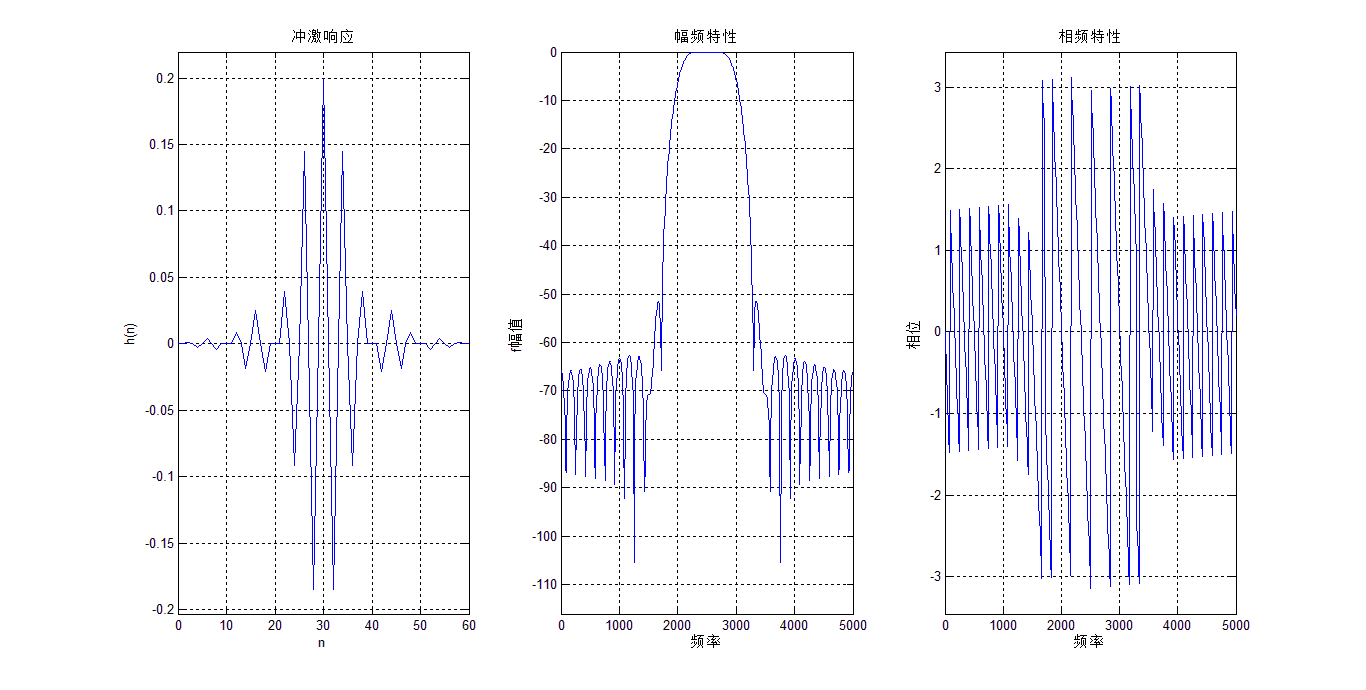展开全文数字信号处理
• FIR滤波器设计文献集-基于MATLAB的FIR滤波器的设计与仿真.pdf 本帖最后由 zyzhang 于 2012-4-24 18:52 编辑 载自各大数据库希望能帮到大家 基于Matlab的FIR带通滤波器的设计与仿真.pdf 基于...matlab
• MATLAB设计FIR滤波器方法程序分享-FIR滤波器设计.doc 里面有低通，高通，带通滤波器的详细设计方法以及程序设计示例，希望对大家有帮助！matlab
• FIR滤波器设计文献集-基于MATLAB的频率采样法设计FIR滤波器.pdf 本帖最后由 zyzhang 于 2012-4-24 18:52 编辑 载自各大数据库希望能帮到大家 基于Matlab的FIR带通滤波器的设计与仿真.pdf 基于...matlab
• FIR滤波器设计文献集-基于Matlab的FIR滤波器在DSP中的实现.pdf 本帖最后由 zyzhang 于 2012-4-24 18:52 编辑 载自各大数据库希望能帮到大家 基于Matlab的FIR带通滤波器的设计与仿真.pdf 基于...matlab
• [Matlab]FIR滤波器设计：(FIR滤波器的结构) FIR(Finite Impulse Response)滤波器：有限长单位冲激响应滤波器，又称为非递归型滤波器，是一种在数字信号领域应用非常广泛的基础型滤波器。FIR滤波器的特点是能够在输入...
[Matlab]FIR滤波器设计：(FIR滤波器的结构)
FIR(Finite Impulse Response)滤波器：有限长单位冲激响应滤波器，又称为非递归型滤波器，是一种在数字信号领域应用非常广泛的基础型滤波器。FIR滤波器的特点是能够在输入具体任意幅频特性的数字信号后，保证输出数字信号的相频特性仍然保持严格线性。另外FIR滤波器是数字信号处理系统中最基本的元件，它可以在保证任意幅频特性的同时具有严格的线性相频特性，同时具有有限长的脉冲采样响应特性，因而滤波器是稳定的系统。因此，FIR滤波器的应用要远胜于IIR滤波器，在通信、图像处理、模式识别等领域都有着广泛而举足轻重的作用。
FIR滤波器的结构：
​ 尽管IIR数字滤波器的设计理论已经非常成熟、经典，但是IIR滤波器有一个自身的中要缺陷：相位特性通常是非线性的。
IIR滤波器的非线性原理：在IIR滤波器的滤波器设计中，只是对滤波器的幅频特性进行了研究，并获得了良好的幅频特性。但对相频特性却没有考虑，所以IIR滤波器的相频特性通常是非线性的。IIR滤波器的应用问题：由于IIR滤波器的相位特性通常是非线性的，所以它适合用于在对系统相位特性要求不严格的场合。但是另一方面，由于一个具有线性相位特性的滤波器可以保证在滤波器通带内信号传输不失真，所以在许多领域需要滤波器具有严格得线性相位特性，比如图像处理以及数据传输等。但是如果要使用IIR滤波器实现线性的相位特性，则必须对其相位特性用全通滤波器进行校正，其结果使得滤波器设计变得非常复杂，实现困难成本提高。所以，要实现具有线性相位的数字滤波器，必须另辟蹊径。有限脉冲响应系统的单位脉冲响应

h

(

s

)

h(s)

为有限长序列，系统函数

H

(

z

)

H(z)

在有限z平面上不存在几点，其运算结构中没有反馈支路，即没有环路。所以，有限脉冲响应滤波器可以设计成在整个频率范围内均可以提供精确的线性相位，而且总是可以独立于滤波器系数保持有限长输入有限长输出的稳定。因此，这样的滤波器在很多领域是首选的。
FIR滤波器的特点
有限长单位冲激响应（FIR）滤波器有以下特点：
系统的单位冲激响应h (n)在有限个n值处不为零系统函数H(z)在|z|>0处收敛，极点全部在z = 0处（因果系统）结构上主要是非递归结构，没有输出到输入的反馈
FIR滤波器的基本结构：
直接型: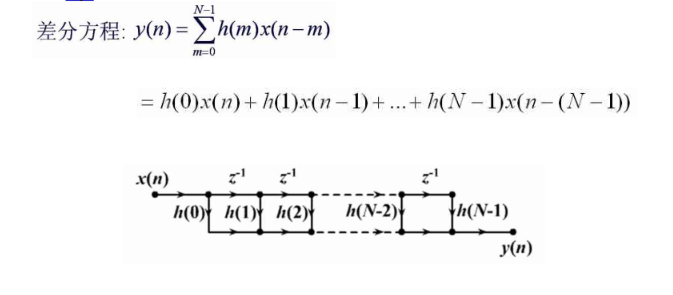级联型: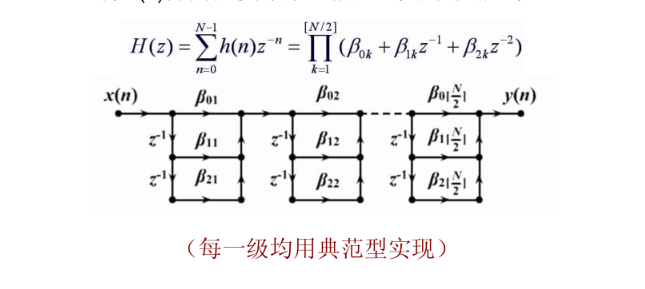特点：
每个基本节控制一对零点便于控制滤波器的传输零点系数比直接型多所需的乘法运算多。
直接型与级联型：
clear all;
n=0:10;
N=30;
b=0.9.^n;
delta=impseq(0,0,N);%脉冲系列生成器
h=filter(b,1,delta);%其中b是分子系数向量，a是分母系数向量。
x=[ones(1,5),zeros(1,N-5)];
y=filter(b,1,x);
subplot(2,2,1);stem(h);%绘制火柴图
title('直接型h(n)');
subplot(2,2,2);stem(y);
title('直接型y(n)');
[b0,B,A]=dir2cas(b,1);
h=casfilter(b0,B,A,delta);%级联型单位脉冲响应
y=casfilter(b0,B,A,x);
subplot(2,2,3);stem(h);
title('级联型h(n)');
subplot(2,2,4);stem(y);
title('级联型y(n)');

%%impseq函数设计生成m文件放入当前文件夹：
function [x,n] = impseq(n0,n1,n2)
% Generates x(n) = delta(n-n0); n1 <= n,n0 <= n2
% ----------------------------------------------
% [x,n] = impseq(n0,n1,n2)
%
if ((n0 < n1) | (n0 > n2) | (n1 > n2))
error('arguments must satisfy n1 <= n0 <= n2')
end
n = [n1:n2];
%x = [zeros(1,(n0-n1)), 1, zeros(1,(n2-n0))];
x = [(n-n0) == 0];

%%dir2cas函数设计生成m文件放入当前文件夹：
function [b0,B,A] = dir2cas(b,a)
% 直接型到级联型的型式转换(复数对型)
% [b0,B,A] = dir2cas(b,a)
% b = 直接型的分子多项式系数
% a = 直接型的分母多项式系数
% b0 = 增益系数
% B = 包含各bk的K乘3维实系数矩阵
% A = 包含各ak的K乘3维实系数矩阵
% compute gain coefficient b0
b0 = b(1); b = b/b0;
a0 = a(1); a = a/a0;
b0 = b0/a0;
%
M = length(b); N = length(a);
if N > M
b = [b zeros(1,N-M)];
elseif M > N
a = [a zeros(1,M-N)]; N = M;
else
NM = 0;
end
%
K = floor(N/2); B = zeros(K,3); A = zeros(K,3);
if K*2 == N;
b = [b 0];
a = [a 0];
end
%
broots = cplxpair(roots(b));
aroots = cplxpair(roots(a));
for i=1:2:2*K
Brow = broots(i:1:i+1,:);
Brow = real(poly(Brow));
B(fix((i+1)/2),:) = Brow;
Arow = aroots(i:1:i+1,:);
Arow = real(poly(Arow));
A(fix((i+1)/2),:) = Arow;
end

%%casfilter函数设计生成m文件放入当前文件夹：
function y = casfilter(b0,B,A,x)
% CASCADE form realization of IIR and FIR filters
% -----------------------------------------------
% y = casfiltr(b0,B,A,x);
%  y = output sequence
% b0 = gain coefficient of CASCADE form
%  B = K by 3 matrix of real coefficients containing bk's
%  A = K by 3 matrix of real coefficients containing ak's
%  x = input sequence
%
[K,L] = size(B);
N = length(x);
w = zeros(K+1,N);
w(1,:) = x;
for i = 1:1:K
w(i+1,:) = filter(B(i,:),A(i,:),w(i,:));
end
y = b0*w(K+1,:);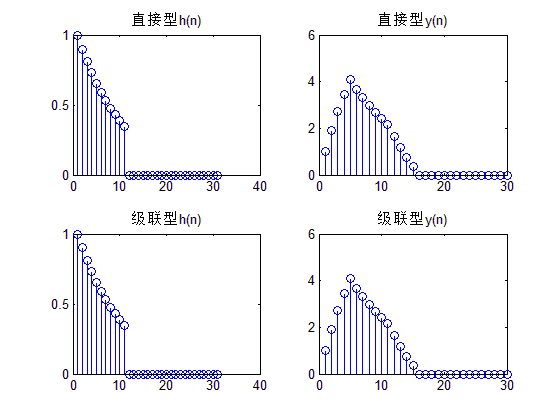快速卷积型: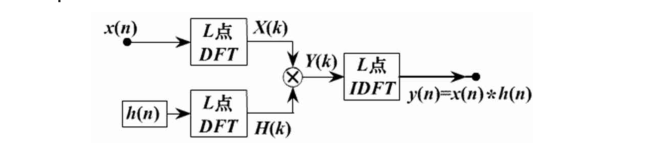运用FFT实现有限长序列

x

(

n

)

x(n)

与

h

(

x

)

h(x)

的线性卷积，则可以得到FIR滤波器的快速卷积结构如图所示，对于

x

(

n

)

x(n)

的无限长序列的一般情况，可以采用重叠相加法或者重叠保留法实现FIR滤波器的快速卷积结构
频率采样型：
FIR系统直接型转换为频率采样型结构的Matlab实现源代码：
function [C,B,A] = dir2fs(h)
% 直接型到频率采样型的转换
% [C,B,A] = dir2fs(h)
% C = 包含各并行部分增益的行向量
% B = 包含按行排列的分子系数矩阵
% A = 包含按行排列的分母系数矩阵
% h =  FIR滤波器的脉冲响应向量
M = length(h);
H = fft(h,M);
magH = abs(H); phaH = angle(H)';
% check even or odd M
if (M == 2*floor(M/2))
L = M/2-1;   %  M为偶数
A1 = [1,-1,0;1,1,0];
C1 = [real(H(1)),real(H(L+2))];
else
L = (M-1)/2; % M is odd
A1 = [1,-1,0];
C1 = [real(H(1))];
end
k = [1:L]';
% 初始化 B 和 A 数组
B = zeros(L,2); A = ones(L,3);
% 计算分母系数
A(1:L,2) = -2*cos(2*pi*k/M); A = [A;A1];
% 计算分子系数
B(1:L,1) = cos(phaH(2:L+1));
B(1:L,2) = -cos(phaH(2:L+1)-(2*pi*k/M));
% 计算增益系数
C = [2*magH(2:L+1),C1]';

案例分析：
%[例6-1]
%%利用频率采样法设计一个低通FIR数字低通滤波器，其理想频率特性是矩形的
%pi=3.14
%通带的截至频率为：w_p = Ωp/f_s = 2*pi Ωp/Ωs = 0.213*pi
%阻带的起始频率为：w_st = Ωst/f_s = 2*pi Ωfs/Ωs = 0.413*pi
%其对应的数字频率：w_c = 2*pi Ωc/Ωs 0.313*pi
%过渡带带宽为：△w = w_st - w_p =0.2*pi  由于△w = (2*pi/N) *3 所以抽样频率为30rad/sec
% 直接型到频率采样型的转换
close all;
clear;
N=30;
H=[ones(1,4),zeros(1,22),ones(1,4)];
H(1,5)=0.5886;H(1,26)=0.5886;H(1,6)=0.1065;H(1,25)=0.1065;
k=0:(N/2-1);k1=(N/2+1):(N-1);k2=0;
A=[exp(-j*pi*k*(N-1)/N),exp(-j*pi*k2*(N-1)/N),exp(j*pi*(N-k1)*(N-1)/N)];
HK=H.*A;
h=ifft(HK);
fs=15000;
[c,f3]=freqz(h,1);
f3=f3/pi*fs/2;
subplot(221);
plot(f3,20*log10(abs(c)));
title('频谱特性');
xlabel('频率/HZ');ylabel('衰减/dB');
grid on;
subplot(222);
title('输入采样波形');
stem(real(h),'.');
line([0,35],[0,0]);
xlabel('n');ylabel('Real(h(n))');
grid on;
t=(0:100)/fs;
W=sin(2*pi*t*750)+sin(2*pi*t*3000)+sin(2*pi*t*6500);
q=filter(h,1,W);
[a,f1]=freqz(W);
f1=f1/pi*fs/2;
[b,f2]=freqz(q);
f2=f2/pi*fs/2;
subplot(223);
plot(f1,abs(a));
title('输入波形频谱图');
xlabel('频率');ylabel('幅度')
grid on;
subplot(224);
plot(f2,abs(b));
title('输出波形频谱图');
xlabel('频率');ylabel('幅度')
grid on;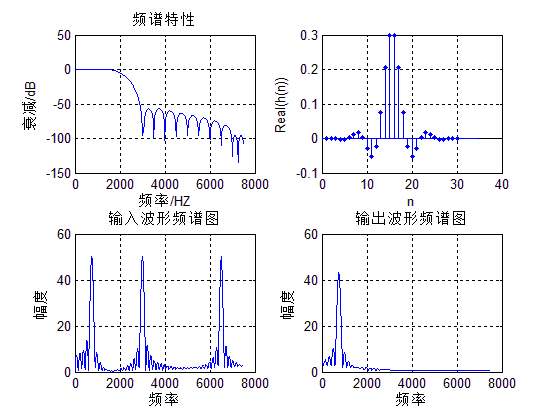展开全文Matlab 数字信号处理
• FIR滤波器设计文献集-基于MATLAB的FIR数字滤波器的设计.pdf 本帖最后由 zyzhang 于 2012-4-24 18:52 编辑 载自各大数据库希望能帮到大家 基于Matlab的FIR带通滤波器的设计与仿真.pdf 基于Matlab...matlab
• FIR滤波器设计文献集-基于频率采样法FIR数字滤波器的设计.pdf 本帖最后由 zyzhang 于 2012-4-24 18:52 编辑 载自各大数据库希望能帮到大家 基于Matlab的FIR带通滤波器的设计与仿真.pdf 基于...matlab
• 《实验二基于语音信号的FIR滤波器设计与实现》由会员分享，可在线阅读，更多相关《实验二基于语音信号的FIR滤波器设计与实现(12页珍藏版)》请在人人文库网上搜索。1、实验二 基于语音信号的FIR滤波器设计与实现一、...

《实验二基于语音信号的FIR滤波器设计与实现》由会员分享，可在线阅读，更多相关《实验二基于语音信号的FIR滤波器设计与实现(12页珍藏版)》请在人人文库网上搜索。
1、实验二 基于语音信号的FIR滤波器设计与实现一、实验目的1. 掌握利用matlab的滤波器设计工具设计FIR滤波器系数2. 熟悉FIR数字滤波器工作原理及其编程设计3. 了解ICETEK-C6713-A板上语音codec芯片TLV320AIC23的设计和程序控制原理4. 掌握分析FIR滤波器滤除噪声与干扰的方法5. 学习使用CCS图形观察窗口观察与分析输入波形及其频谱二、实验设备1PC兼容机一台；操作系统为 WindowsXP 2ICETEK-C6713A-S60实验箱一台。 3USB 连接电缆一条三实验原理1TLV320AIC23 芯片性能指标及控制方法：初始化配置：DSP 通过总线将配置命。
3、Hamming Window)函数，截止频率为7000Hz，采样频率为48000Hz。3程序流程图：四、实验步骤1实验准备：准备连接音频输入、输出设备： 将音频线一端插头插到ICETEK-AIC23板的音频输入口(J4/J5)，另一端插到计算机的音频输出口。 将音频线一端插头插到ICETEK-AIC23板的音频输出口(J6/J7)，另一端插到ICETEK-SG-A板的扬声器输入口。2设置Code Composer Studio 3.3在硬件仿真(Emulator)方式下运行；并启动 Code Composer Studio 3.3；3建立新的工程文件： 工程目录为：E:DSP_LAB12041。
4、102audiofilter4编辑输入程序：供参考的基本代码在E:DSP_LAB实验二FIR_audio目录下(播放的音乐在audio子目录下)，将所有后缀为asm、c、h、cmd的文件复制到E:DSP_LAB12041102目录下。其中的滤波器系数(fHnLFIRNUMBER)和单频干扰系数(noisFIRNUMBER)为空，需要通过matlab进行设计。滤波器设计工具在命令窗口中敲入fdatool即可打开，单频干扰系数可以调用sin函数生成。5编译源文件、下载可执行程序。同实验一。2设置观察窗口，观察滤波效果显示：执行View Watch Window打开观察窗口。 打开 aic23_lo。
5、opback.c，通过“Quick Watch”,将变量 (比如bCode)加入观察窗口。 通过改变bCode的值来实现原始信号(0)/加噪信号(1)/滤波信号(其它值)的输出切换采用实验一的方法，通过CCS内部画图功能，观察中间结果。3运行程序，听效果 使用计算机提供的声源：启动播放语音文件E:DSP_LAB实验二audio子目录下的mp3文件，并选择播放器参数为循环播放;按“F5”键，可以听到立体声线路输入的语音信号。这时的语音信号并没有经过滤波处理。修改观察窗口中 bCode的值为1，此时语音信号中被加入单频噪声；修改观察窗口中 bCode的值为2，此时启动FIR滤波；听效果。反复修改 。
6、bCode的值成 0 ，1，2，比较原声，加载噪声之后的音频以及滤波后的声音。 4通过FDATOOL设计不同类型、通带的滤波器，反复实验上述效果，记录不同滤波器条件下的语言变化。5结束运行，关闭工程，退出 CCS。五、实验结果1通过播放音乐可以明显的区分加噪过程和滤波过程。2通过CCS内部画图功能，可以看到如下结果：图1 原始信号频谱图通过频谱图可以看出原始信号频谱比较集中，在低频和高频处各有两个尖峰，但是在其他位置几乎没有频谱分量，试听过程中，音乐清晰。图2 加噪信号频谱图在加入噪声后，信号在高频部分的分量减少，低频部分的尖峰由一个变为两个，同时在其他位置也出现频谱分量，在试听过程中，也能听。
7、到比较大的刺啦声，效果明显。图3 (a)滤波器频率特性(Hamming窗)(b)过低通滤波器信号频谱图在通过低通滤波器后，我们可以明显的看到高频分量消失，试听过程中部分高音消失，音乐声音变小，但仍能听到声音。图4 (a)滤波器频率特性(Hamming窗)(b)过带通滤波器信号频谱图在通过带通滤波器后，可以明显的看到信号的频率被限制在一定的频带范围内，低频分量和高频分量均被抑制。根据带通滤波器的设计不同，可以将声音限制在更窄的频带范围内。试听过程中表现为声音不够清晰，大部分人声消失。图5 (a)滤波器频率特性(Hamming窗)(b)过高通滤波器信号频谱图在通过高通滤波器后发现信号没有任何变化，。
8、高通滤波器不起作用，反复设计多个高通滤波器结果相同。(c)滤波器频率特性(Hamming窗)(d)过等效高通(带通)滤波器信号频谱图于是，设计了一个对于语音信号来说等效于高通滤波器的带通滤波器，通过实验发现，低频信号被滤掉。原因是：此处计算信号功率谱为实功率谱，功率谱具有镜像对称性，因此，复功率谱部分被对称到高频部分，所以加入高通滤波器相当于滤掉原始功率谱，使镜像功率谱通过，因此仍包含信号的全部分量，等效于没有滤掉任何频谱分量。图6 (a)滤波器频率特性(Hanning窗)(b)过滤波器信号频谱图滤波器采用相同的设计参数，对比滤波器的频率特性，发现Hanning窗比Hamming窗的主瓣宽度要。
9、窄，但旁瓣的抑制率低。表现在实验中可以发现Hanning窗下信号频谱范围比Hamming窗要宽。图7 (a)滤波器频率特性(Blackman窗)(b)过滤波器信号频谱图滤波器采用相同的设计参数，对比滤波器的频率特性，发现Blackman窗比Hamming窗的主瓣宽度要宽，但旁瓣的抑制率高。表现在实验中可以发现Blackman窗下信号频谱范围比Hamming窗要宽许多。但是由于该语音信号频谱比较集中，因此对滤波器性能的验证并不明显。图8 (a)滤波器频率特性(Kaiser窗)(b)过滤波器信号频谱图滤波器采用相同的设计参数，对比滤波器的频率特性，发现Blackman窗比Hamming窗的主瓣宽度要窄许多，但旁瓣的抑制率低。表现在实验中可以发现Kaiser窗下信号主要频率范围比Hamming窗要窄许多，但是频谱比较宽。六实验心得通过本次试验，掌握分析FIR滤波器滤除噪声与干扰的方法，学会使用CCS图形观察窗口观察与分析输入波形及其频谱，同时对不同滤波器的性能有了更加深入的理解。并且在实验过程中，可调参数比较多，需要采集大量的数据，对比不同的实验结果，虽然过程比较烦琐，但是颇有科学研究的感觉，同时能够发下问题并找到原因，将时间与理论相结合。

展开全文• FIR滤波器设计文献集-基于Matlab的FIR带通滤波器的设计与仿真.pdf 本帖最后由 zyzhang 于 2012-4-24 18:52 编辑 载自各大数据库希望能帮到大家 基于Matlab的FIR带通滤波器的设计与仿真.pdf 基于...matlab
• 1引言 数字滤波是数字信号处理的一种重要算法 广泛用于对信号的...无限脉冲响应IIR 滤波器两类FIR 滤波器的线性与稳定性使其应用更为广泛本文主要介绍采用窗函数法设计FIR 滤波器及其MATLAB 的软件实现方法 2FIR 数字滤
• 基于FPGA平台的FIR滤波器实现，结合明德杨实验板及设计思路进行验证
• 基于MATLAB的IIR和FIR滤波器设计-实验4 FIR数字滤波器设计.doc IIR：在MATLAB中，可以用下列函数辅助设计IIR数字滤波器：1)利用buttord和cheb1ord可以确定低通原型巴特沃斯和切比雪夫滤波器的阶数和截止频率；2)...matlab...